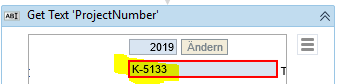# If condition for first letter

Hello together,

i want to do with the following variable a IF conditionif the first letter is an “I” or a “F”, i need only the first six figures in my variable. If not, then i use the whole text. What do i have to enter to geht only the first six figures?

Thanks and Regards
Roberto

Hi,

Try this Variable.Substring(0,6)

Hi,

FYI, you can get it using following expression without IF activity.

`System.Text.RegularExpressions.Regex.Match(strData,"(?(^[IF])^.{6}|^.*)").Value`

Regards,

Thanks all, with the if condition it works. But just to have other options, were should i enter this
System.Text.RegularExpressions.Regex.Match(strData,“(?()^.{6}|^.*)”).Value

Thanks and Regards
Roberto

1. IF ↩︎

1 Like

Use
If — Variable.Substring(0,1)=“I” OR Variable.Substring(0,1)=“K”
Then
NewVar=Variable.Substring(0,6)
else
NewVar=Variable